How to Calculate and Solve for Poisson’s Ratio | Rock Mechanics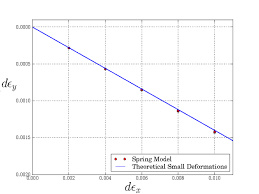The image above represents Poisson’s ratio.

To compute for Poisson’s ratio, two essential parameters are needed and these parameters are lateral strain (εland axial strain (εa).

The formula for calculating Poisson’s ratio:

v = εl / εa

Where:

v = Poisson’s Ratio
εl = Lateral Strain
εa = Axial Strain

Let’s solve an example;
Find the Poisson’s ratio when the lateral strain is 80 and the axial strain is 4.

This implies that;

εl = Lateral Strain = 80
εa = Axial Strain = 4

v = εl / εa
v = 80 / 4
v = 20

Therefore, the Poisson’s ratio is 20.

Calculating the Lateral Strain when the Poisson’s Ratio and Axial Strain is Given.

εl = v x εa

Where:

εl = Lateral Strain
v = Poisson’s Ratio
εa = Axial Strain

Let’s solve an example;
Find the lateral strain when the Poisson’s ratio is 40 and the axial strain is 9.

This implies that;

v = Poisson’s Ratio = 40
εa = Axial Strain = 9

εl = v x εa
εl = 40 x 9
εl = 360

Therefore, the lateral strain is 360.

Calculating the Axial Strain when the Poisson’s Ratio and Lateral Strain is Given.

εa  = εl / v

Where:

εa = Axial Strain
v = Poisson’s Ratio
εl = Lateral Strain

Let’s solve an example;
Find the axial strain when the Poisson’s ratio is 20 and the lateral strain is 120.

This implies that;

v = Poisson’s Ratio = 20
εl = Lateral Strain = 120

εa = εl / v
εa = 120 / 20
εa = 60

Therefore, the axial strain is 60.

Nickzom Calculator – The Calculator Encyclopedia is capable of calculating the Poisson’s ratio.

To get the answer and workings of the Poisson’s ratio using the Nickzom Calculator – The Calculator Encyclopedia. First, you need to obtain the app.

You can get this app via any of these means:

To get access to the professional version via web, you need to register and subscribe for NGN 1,500 per annum to have utter access to all functionalities.
You can also try the demo version via https://www.nickzom.org/calculator

Android (Paid) – https://play.google.com/store/apps/details?id=org.nickzom.nickzomcalculator
Android (Free) – https://play.google.com/store/apps/details?id=com.nickzom.nickzomcalculator
Apple (Paid) – https://itunes.apple.com/us/app/nickzom-calculator/id1331162702?mt=8
Once, you have obtained the calculator encyclopedia app, proceed to the Calculator Map, then click on Geology under Add-on.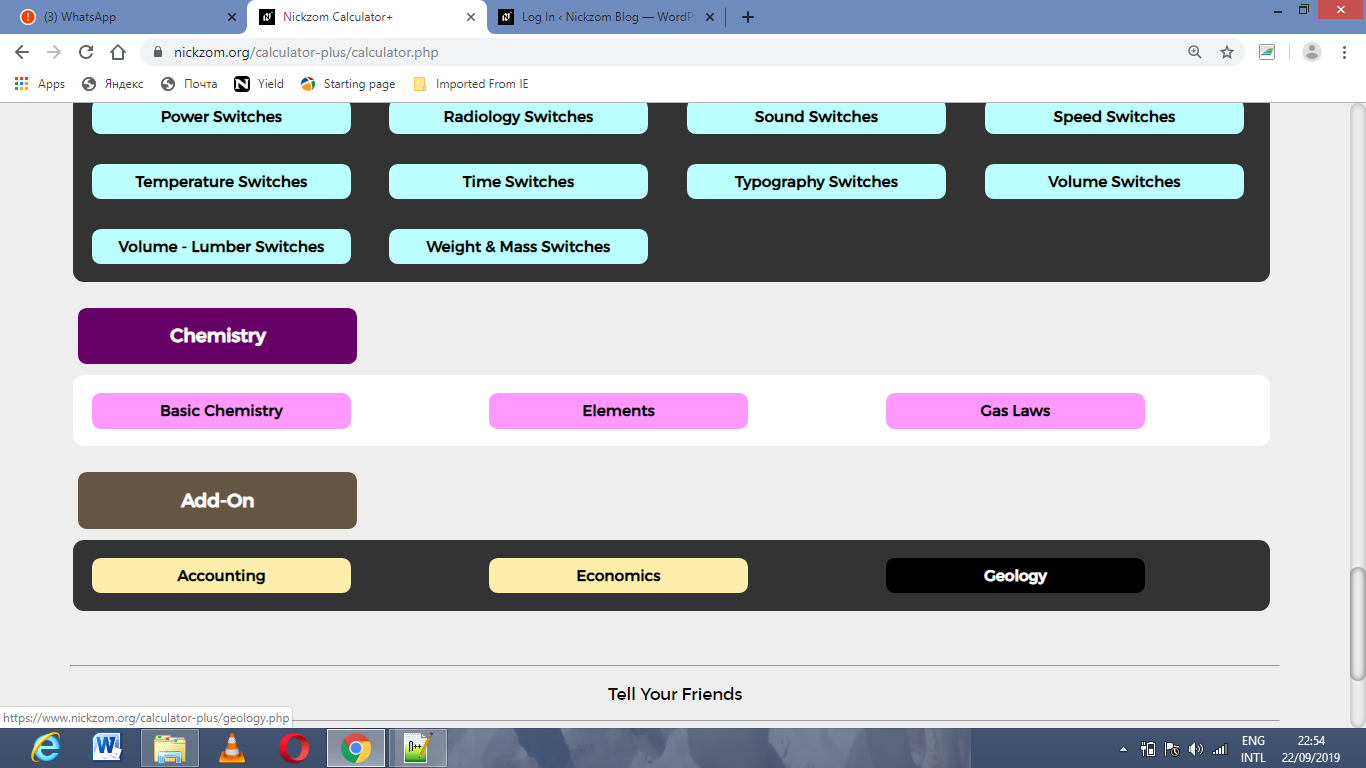Now, Click on Rock Mechanics under GeologyNow, Click on Poisson’s Ratio under Rock MechanicsThe screenshot below displays the page or activity to enter your values, to get the answer for the Poisson’s ratio according to the respective parameters which are the lateral strain (εl) and axial strain (εa).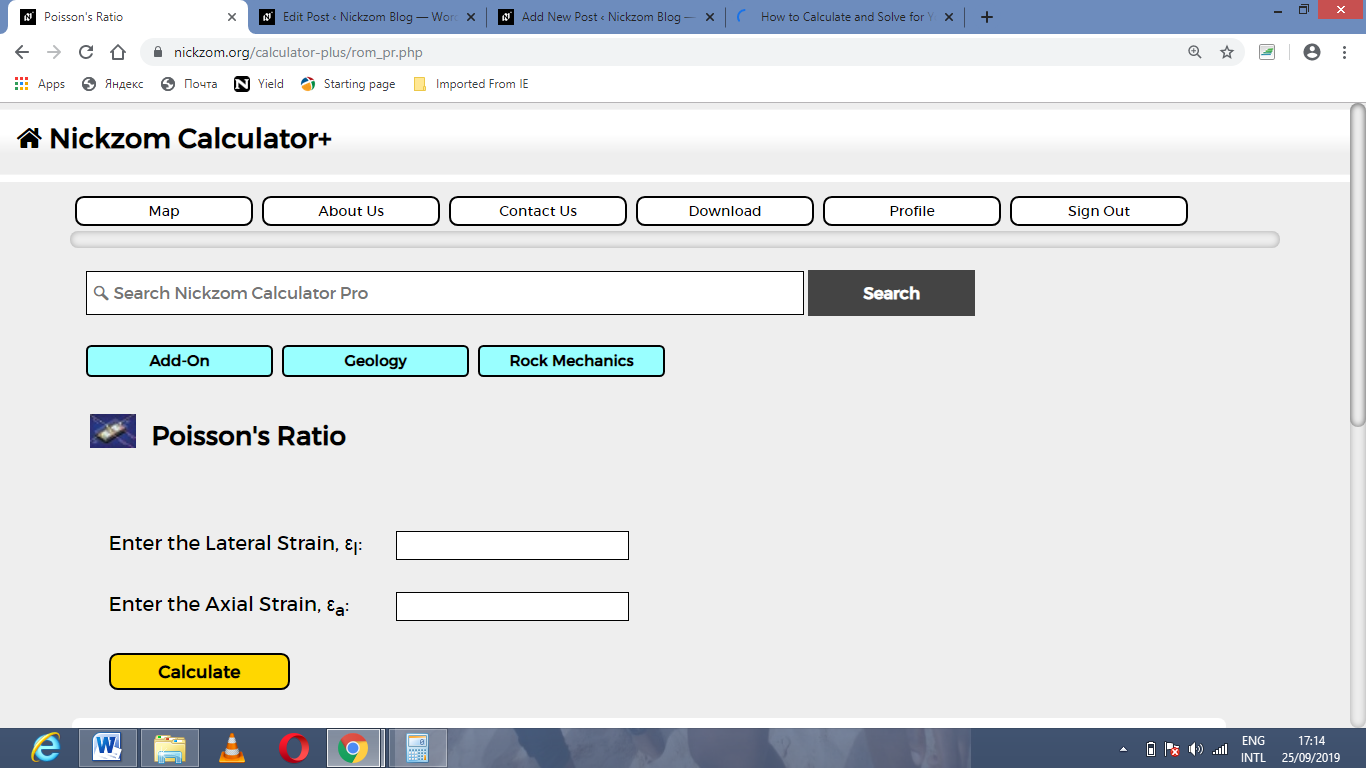Now, enter the values appropriately and accordingly for the parameters as required by the lateral strain (εl) is 80 and axial strain (εa) is 4.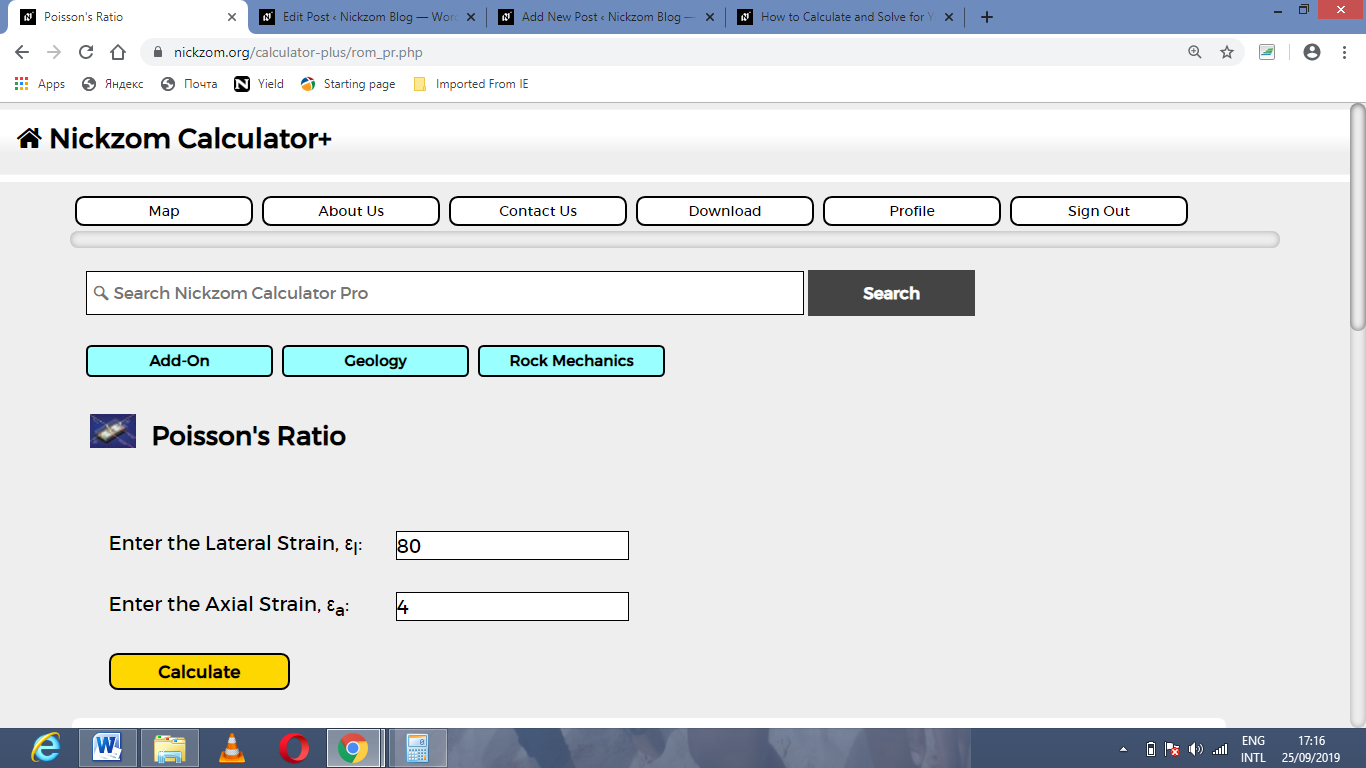Finally, Click on Calculate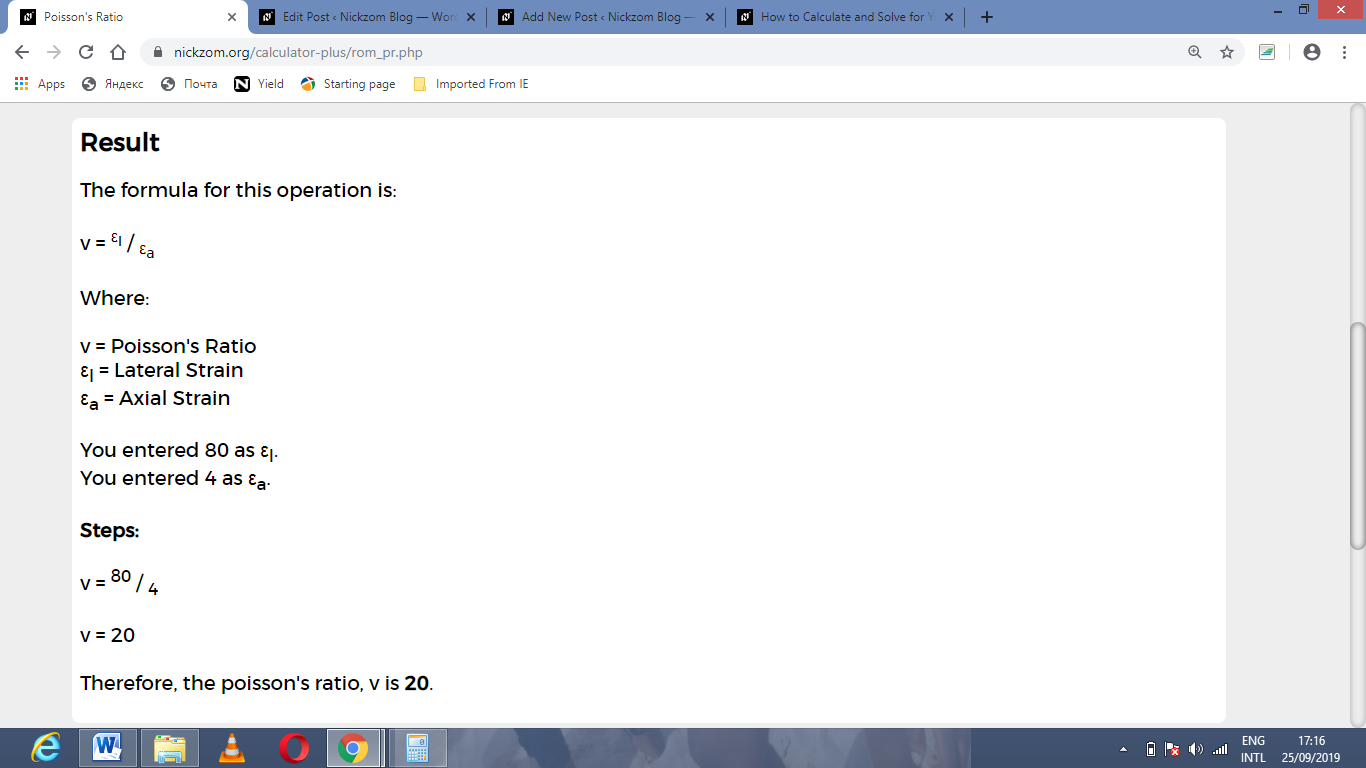As you can see from the screenshot above, Nickzom Calculator– The Calculator Encyclopedia solves for the Poisson’s ratio and presents the formula, workings and steps too.